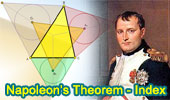# Math: Plane Geometry: Napoleon's Theorem, College, High School

 Napoleon's Theorem, IndexGeometry Problem 1205 Triangle, Centroid, Outer and Inner Napoleon Equilateral Triangles. Proposed Problem 248. Napoleon's Theorem III. Inner and outer Napoleon triangles, Area. Proposed Problem 247. Napoleon's Theorem II. Internal Equilateral triangles. Inner Napoleon triangle. Proposed Problem 246. Napoleon's Theorem I. External Equilateral triangles. Napoleon's Theorem. A purely geometric proof. It uses the Fermat point to prove Napoleon without transformations. Proposed Problem 404. External Equilateral triangles, Congruent and Concurrent Lines. (Fermat Point) Proposed Problem 243. Triangle with Equilateral triangles, Parallelogram. Proposed Problem 242. Triangle with Equilateral triangles, Parallelogram. Proposed Problem 241. Triangle with Equilateral triangles, Congruence. Proposed Problem 240. Triangle with Equilateral triangles, Parallelogram. Proposed Problem 50. Triangle with Equilateral triangles. Seventeen conclusions. Geometry Problem 1125. Triangle, Four Equilateral Triangles, Centroid, Midpoint.

 Home | Search | Geometry | Triangles | Post a comment | Email | By Antonio Gutierrez Last updated: Apr 9, 2016